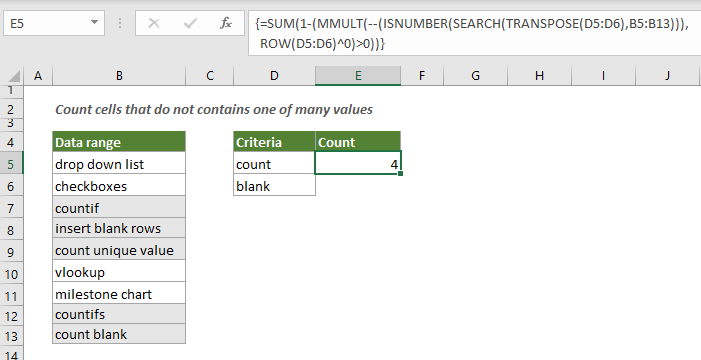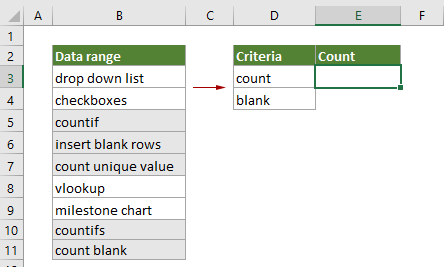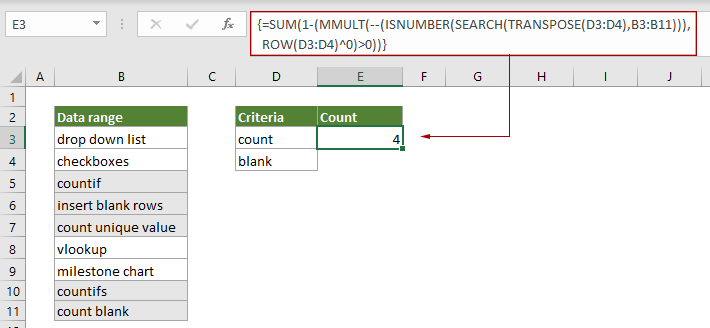## Count number of cells that do not contain many values

Normally, it is easy to count cells that do not contain one specific value with the COUNTIF function. This post will step through how to count number of cells that do not contain many values in a specified range in Excel.#### How to count cells that do not contain many values?

As the below screenshot shown, to count cells in B3:B11 that do not contain values listed in D3:D4, you can do as follows.Generic Formula

{=SUM(1-(MMULT(--(ISNUMBER(SEARCH(TRANSPOSE(criteria_range),range))),ROW(criteria_range)^0)>0))}

Arguments

Range (required): The range from which you want to count cells that do not contain many values.

Criteria_range (required): The range contains the values you want to exclude when counting cells.

Note: This formula must be entered as an array formula. After applying the formula, if there are curly brackets wrapped around the formula, an array formula is successfully created.

How to use this formula?

1. .Select a blank cell to output the result.

2. Enter the below formula into it and then press the Ctrl + Shift + Enter keys simultaneously to get the result.

=SUM(1-(MMULT(--(ISNUMBER(SEARCH(TRANSPOSE(D3:D4),B3:B11))),ROW(D3:D4)^0)>0))How these formulas work?

=SUM(1-(MMULT(--(ISNUMBER(SEARCH(TRANSPOSE(D3:D4),B3:B11))),ROW(D3:D4)^0)>0))

1) --(ISNUMBER(SEARCH(TRANSPOSE(D3:D4),B3:B11))):

• TRANSPOSE(D3:D4):The TRANSPOSE function rotates the orientation of D3:D4 and returns {“count”,”blank”};
• SEARCH({“count”,”blank”},B3:B11):The SEARCH function here finds the position of substring “count” and “blank” from range B3:B11, and returns an array as {#VALUE!,#VALUE!;#VALUE!,#VALUE!;1,#VALUE!;#VALUE!,8;1,#VALUE!;#VALUE!,#VALUE!;#VALUE!,#VALUE!;1,
#VALUE!;1,7}.
• In this case, each cell in B3:B11 will be searched twice as there are two values you want to exclude when counting cells, so you will get 18 values in the array. Each number in the array indicates the position of the first character of “count” or “blank” in each cell of B3:B11.
• ISNUMBER{#VALUE!,#VALUE!;#VALUE!,#VALUE!;1,#VALUE!;#VALUE!,8;1,#VALUE!;#VALUE!,#VALUE!;#VALUE!,
#VALUE!;1,#VALUE!;1,7}
:The ISNUMBER function returns TRUE if meeting numbers in the array, and returns FALSE if meeting errors. Here returns the result as {FALSE,FALSE;FALSE,FALSE;TRUE,FALSE;FALSE,TRUE;TRUE,FALSE;FALSE,FALSE;FALSE,FALSE;TRUE,FALSE;
TRUE,TRUE}.
• --({FALSE,FALSE;FALSE,FALSE;TRUE,FALSE;FALSE,TRUE;TRUE,FALSE;FALSE,FALSE;FALSE,FALSE;TRUE,
FALSE;TRUE,TRUE})
:These two minus signs convert “TRUE” into 1 and convert “FALSE” into 0. Here you will get a new array as {0,0;0,0;1,0;0,1;1,0;0,0;0,0;1,0;1,1}.

2) ROW(D3:D4)^0:The ROW function returns the row numbers of the cell reference: {3;4}, and then the exponentiation operator (^) returns the result of the number 3 and 4 raised to a power 0, and finally returns the result as {1;1}.

3) MMULT({0,0;0,0;1,0;0,1;1,0;0,0;0,0;1,0;1,1},{1;1}):The MMULT function returns the matrix product of these two arrays: {0;0;1;1;1;0;0;1;2} to meet the original data. Any non-zero number in the array represents a value where at least one of the excluded strings has been found, and zero indicates no excluded strings were found.

4) SUM(1-{0;0;1;1;1;0;0;1;2}>0):

• {0;0;1;1;1;0;0;1;2}>0:Here check if each number in the array is greater than 0. If a number is greater than 0, returns TRUE, if not, returns FALSE. Then you will get a new array as {FALSE;FALSE;TRUE;TRUE;TRUE;FALSE,FALSE,TRUE;TRUE}.
• 1-{FALSE;FALSE;TRUE;TRUE;TRUE;FALSE,FALSE,TRUE;TRUE}:As we need to count only the cells that do not contain the specified values, we need to reverse these values in the array by subtracting them from 1. Here the math operator automatically converts TRUE and FALSE values to 1s and 0s, and finally returns the result as {1;1;0;0;0;1;1;0;0}.
• SUM{1;1;0;0;0;1;1;0;0}:The SUM function sums all numbers in the array and returns the final result as 4.

#### Related functions

Excel SUM function
The Excel SUM function adds values.

Excel MMULT function
The Excel MMULT function returns the matrix product of two arrays.

Excel ISNUMBER function
The Excel ISNUMBER function returns TRUE when a cell contains a number, and FALSE if not.

Excel TRANSPOSE function
The Excel TRANSPOSE function rotates the orientation of a range or array.

Excel ROW function
The Excel ROW function returns the row number of a reference.

#### Related formulas

Count cells that do not contain errors
This tutorial provides detailed steps to help you count the number of cells that do not contain errors in a specified range in Excel.

Count Cells That Do Not Contain Specific Text
You can use the COUNTIF function with a wildcard to count the number of cells that contain specific text in a range. On the contrary, it is also easy to use the COUNTIF function to count cells that do not contain specific text. This tutorial provides detailed steps to help solving the problem.

Count number of date cells in a range by day of week
Excel provides the combination of SUMPRODUCT and WEEKDAY functions to help us easily count the number of specified weekdays within a range. This tutorial provides a step by step guide to help you get through it.

Count The Number Of Text Cells
To count the number of cells that contain text in a specified range, the COUNTIF function can help to easily get it done. Here this article will introduce the formula in details to help you solve the problem.

### The Best Office Productivity Tools

#### Kutools for Excel - Helps You To Stand Out From Crowd

 Popular Features: Find, Highlight or Identify Duplicates  |  Delete Blank Rows  |  Combine Columns or Cells without Losing Data  |  Round without Formula ... Super VLookup: Multiple Criteria  |  Multiple Value  |  Across Multi-Sheets  |  Fuzzy Lookup... Adv. Drop-down List: Easy Drop Down List  |  Dependent Drop Down List  |  Multi-select Drop Down List... Column Manager: Add a Specific Number of Columns  |  Move Columns  |  Toggle Visibility Status of Hidden Columns  |  Compare Columns to Select Same & Different Cells ... Featured Features: Grid Focus  |  Design View  |  Big Formula Bar  |  Workbook & Sheet Manager | Resource Library (Auto Text)  |  Date Picker  |  Combine Worksheets  |  Encrypt/Decrypt Cells  |  Send Emails by List  |  Super Filter  |  Special Filter (filter bold/italic/strikethrough...) ... Top 15 Toolsets:  12 Text Tools (Add Text, Remove Characters ...)  |  50+ Chart Types (Gantt Chart ...)  |  40+ Practical Formulas (Calculate age based on birthday ...)  |  19 Insertion Tools (Insert QR Code, Insert Picture from Path ...)  |  12 Conversion Tools (Numbers to Words, Currency Conversion ...)  |  7 Merge & Split Tools (Advanced Combine Rows, Split Excel Cells ...)  |  ... and more

Kutools for Excel Boasts Over 300 Features, Ensuring That What You Need is Just A Click Away...#### Office Tab - Enable Tabbed Reading and Editing in Microsoft Office (include Excel)

• One second to switch between dozens of open documents!
• Reduce hundreds of mouse clicks for you every day, say goodbye to mouse hand.
• Increases your productivity by 50% when viewing and editing multiple documents.
• Brings Efficient Tabs to Office (include Excel), Just Like Chrome, Edge and Firefox.